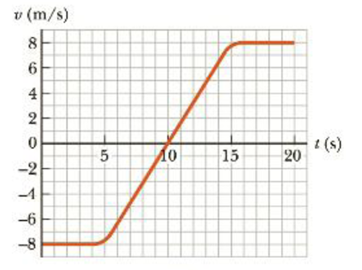Chapter 2, Problem 24P

Chapter
Section
Textbook Problem

The velocity vs. time graph for an object moving along a straight path is shown in Figure P2.21. (i) Find the average acceleration of the object during the time intervals (a) 0 to 5.0 s, (b) 5.0 s to 15 s. and (c) 0 to 20 s. (ii) Find the instantaneous acceleration at (a) 2.0 s, (b) 10 s, and (c) 18 s.Figure P2.24

(i)

(a)

To determine
The average acceleration of the object.

Explanation

Given info:

The initial velocity of the object is 8.0m/s .

The final velocity of the object is 8.0m/s .

The initial time of the interval is 0 .

The final time of the interval is 5.0s .

Formula to calculate average acceleration is,

a¯=vfvitfti

• a¯ is the average acceleration of the object
• vf is the final velocity of the object
• vi is the initial velocity of the object
• ti final time of the interval
• tf final time of the interval

Substitute 8

(b)

To determine
The average acceleration of the object.

(c)

To determine
The average acceleration of the object.

(ii)

(a)

To determine
The instantaneous acceleration of the object at 2.0s .

(b)

To determine
The instantaneous acceleration of the object at 10s .

(c)

To determine
The instantaneous acceleration of the object at 18s .

Still sussing out bartleby?

Check out a sample textbook solution.

See a sample solution

The Solution to Your Study Problems

Bartleby provides explanations to thousands of textbook problems written by our experts, many with advanced degrees!

Get Started